Next: About this document ... Up: lab_template Previous: lab_template

Subsections

# MA 1024A Laboratory 3: Surfaces

## Purpose

The purpose of this lab is to introduce you to some of the Maple commands that can be used to plot surfaces in three dimensions.

## Background

The graph of a function of a single real variable is a set of points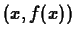in the plane. Typically, the graph of such a function is a curve. For functions of two variables, the graph is a set of points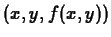in three-dimensional space. For this reason, visualizing functions of two variables is usually more difficult.

One of the most valuable services provided by computer software such as Maple is that it allows us to produce intricate graphs with a minimum of effort on our part. This becomes especially apparent when it comes to functions of two variables, because there are many more computations required to produce one graph, yet Maple performs all these computations with only a little guidance from the user.

Two common ways of representing the graph of a function of two variables are the surface plot and the contour plot. The first is simply a representation of the graph in three-dimensional space. The second, draws the level curves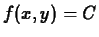for several values ofin the-plane. We will explore how to produce these kinds of graphs in Maple, and how to use the graphs to study the functions.

### Cartesian coordinates

You can define functions of more than one variable in much the same way as you defined functions of a single variable:

> f := (x,y) -> x^2 + y^2;
> f(3,-1);
> g := (a,b,c,d,e) -> a*b^2 - sin(c+d)/e;


The following commands are useful for working with functions of two variables in Maple.

#### plot3d

This command generates a surface plot of the function. This command has a lot of options that you can use to get the plot to look the way you want it. Some of the most useful options are described below. For more information, see the help for the plot3d command.
> plot3d(x^2-y^2, x=-1..1, y=-1..1);
> f := (x,y) -> x/2 - y + 3;
> plot3d(f, 0..2, -1..1);

The default viewing angle is from a direction 45 degrees between the positive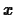- and positive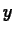- axes, and an angle of elevation of 45 degrees. You can change this viewing angle with the orientation option, orientation=[a,b]. The first number is the polar angle, measured counterclockwise from the positive-axis. The second number is the angle of elevation; it is measured downward from straight above, also in degrees.

You can also select a viewpoint using the mouse. Click the mouse on a three-dimensional graph, and notice the context bar that appears between the tool bar and the Maple input/output window. Click the graphic again, and the graph is replaced by a box. Hold down the button as you move the mouse, and you'll see the box from different angles. You'll also see the numbers on the left, labeledand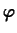, change accordingly. They correspond to the two numbers in the orientation option.

Once you've selected the desired viewpoint, redraw the graphic by pressing the button marked R' at the right end of the tool bar.

The other buttons in the tool bar control other aspects of how the plot is drawn, including the plot style, axes style, etc.

The number of grid points in the plot can be changed with the grid=[x,y] option. You may want to increase the number of grid points if your plot appears rough, or has a lot of oscillation; you may want to use a smaller number if the function is reasonably smooth and you want to shorten calculation times.

> plot3d(x^2-y^2,x=-1..1,y=-1..1,orientation=[45,45]);
> plot3d(x^2-y^2,x=-1..1,y=-1..1,orientation=[45,20]);
> plot3d(x^2-y^2,x=-1..1,y=-1..1,orientation=[45,-45]);
> plot3d(x^2-y^2,x=-1..1,y=-1..1,orientation=[110,45]);
> plot3d(x^2-y^2,x=-1..1,y=-1..1,grid=[10,10]);
> plot3d(x^2-y^2,x=-1..1,y=-1..1,grid=[25,40]);

For more information on options, you can type ?plot3d,option.

Note that you can use the context bar instead of optional arguments to the plot3d command to customize your plot. Saving your worksheet after you have made changes will save the plot as it last appeared. However, if you need to run the plot3d command again, any customizations you made with the context bar will be lost. The safest approach is to use the context bar to experiment with your plot until you are satisfied with it. Then add options to your plot3d command that will give you the same plot, for example by specifying the orientation or axes arguments in your command.

#### contourplot

Generates a contour plot of a function of two variables. This command is part of the plots'' package, so you need to run with(plots) before using the command. The basic syntax is the same as for plot3d.
> with(plots):
> contourplot(x^2-y^2,x=-1..1,y=-1..1);
> f := (x,y) -> x/2 - y + 3;
> contourplot(f,0..2,-1..1);

Maple's default is to produce ten contours. This can be changed using the option contours=n. Maple chooses the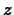-levels of the contours automatically. If you want to see specific level curves, you can write the-values in a list.
> contourplot(x^2-y^2,x=-2..2,y=-2..2);
> contourplot(x^2-y^2,x=-2..2,y=-2..2,contours=6);
> contourplot(x^2-y^2,x=-2..2,y=-2..2,contours=);
> contourplot(x^2-y^2,x=-2..2,y=-2..2,contours=[-1,0,1,2]);


#### implicitplot3d

If a surface cannot be easily written asbut can be written as a relation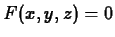, then the Maple implicitplot3d command from the plots package can often be used. For example, to plot the cylinder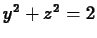the following command can be used. Don't forget to load the plots package first using the command with(plots); first.
> implicitplot3d(y^2+z^2=2,x=0..2,y=-2..2,z=-2..2);

Getting a good plot using this command can be a little tricky because you have to come up with good guesses for the ranges for,, and. If most or all of the surface is outside the ranges you specify, you may not see a good representation of the surface.

### Cylindrical and spherical coordinates

Maple can also do plots of surfaces in cylindrical and spherical coordinates. To do this, you just use the coords option to the plot3d command to specify the coordinate system. For example, the following command will plot the cylinder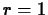for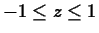.
> plot3d(1,theta=0..2*Pi,z=-1..1,coords=cylindrical);

The next command plots the cone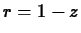over the same range for.
> plot3d(1-z,theta=0..2*Pi,z=-1..1,coords=cylindrical);

The plot3d command expects your equation for the surface to be of the form, withthe first independent variable andthe second independent variable. If your equation isn't of this form, then you have to use a parametric plot. Parametric plots of surfaces are usually beyond the scope of this course, but we will present an example in Cartesian coordinates at the end of this section.

Plotting a surface in spherical coordinates is very similar. Maple expects the equation of the surface to be in the form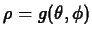. Again, the order is important. The following command plots the unit sphere.

> plot3d(1,theta=0..2*Pi,phi=0..Pi,coords=spherical);

Many surfaces can be represented in more than one coordinate system. For example, the following command plots the same cylinder of radius 1 that we plotted before, but in spherical coordinates.
> plot3d(1/sin(phi),theta=0..2*Pi,phi=Pi/4..3*Pi/4,coords=spherical);


The implicitplot3d command can also be used to plot relations in terms of cylindrical or spherical coordinates. You just use the same coords optional argument that you used for the plot3d command. The only tricky part is that it expects you to give the ranges in a certain order. For example, in cylindrical coordinates it expects the range for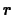first, then the range for, and finally the range for. Other orders can give unpredictable results. For spherical coordinates, the order must be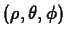.

### Parametric surfaces

Sometimes a surface cannot easily be represented in Cartesian, polar, or even spherical coordinates. In these cases, a parametric plot of the surface is often used. We have already seen parametric curves, in which a single parameter, usually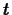was used. For a surface, two parameters are required. A parametric surface in Cartesian coordinates is an ordered triple of functions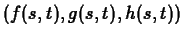where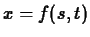,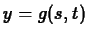, and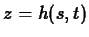. That is, given values of the parameters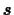andyou can compute a point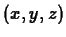on the surface. For example, any surface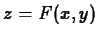can be represented parametrically as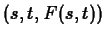. However, the real utility of a parametric surface is for surfaces that cannot be represented asor even as a simple relation between,, and. For example suppose you wanted to plot the cylinderfor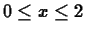. You could solve for, but that gives the two functionsand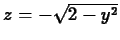and you would have to combine them to plot the cylinder. An alternative is the following.
> plot3d([s,2*cos(t),2*sin(t)],s=0..2,t=0..2*Pi);

We used the implicitplt3d command above to plot this same cylinder. An advantage of using a parametric plot is that you usually know what ranges to use for your parameters to get the plot you want.

As a final example of a parametric surface, we present the torus, or doughnut if you are feeling hungry.

> plot3d([4*cos(s)+cos(t)*cos(s),4*sin(s)+cos(t)*sin(s),sin(t)],
s=0..2*Pi,t=0..2*Pi,style=patch);
`

### Printing problems

There are occasionally problems printing out a Maple worksheet that has many three dimensional plots. The usual symptom is that part of the worksheet is not printed. If this happens to you, the best method for overcoming this problem in the past has been to delete all of the output in your worksheet, save it, and then re-execute the whole worksheet before attempting to print again. The best way to delete all output is to use the Remove Output item from the Edit menu. There is also an Execute option in the Edit menu that you can use to execute all the commands in your worksheet. If this doesn't work, consult your instructor.

The unfortunate thing about this problem is that modifications you might have made to your plots using the mouse or the context bar are lost. This is why it is a good idea to first experiment with your plot using the mouse and the context bar but, once you have the plot looking the way you want it, to include your modifications in the plot command.

## Exercises

1. Generate a surface plot and contour plot for each of the following functions on the given domains:
1.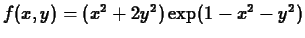, forand.

2.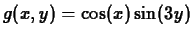, forand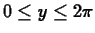.
2. What does the contour plot look like in the regions where the surface plot has a steep incline? What does it look like where the surface plot is almost flat?
3. What can you say about the surface plot in a region where the contour plot looks like a series of nested circles?

1. Can you distinguish between the contour plots for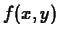and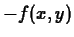? If so, how? If not, how would you overcome this problem?

2. Generate a surface plot for the following functions on the domains given.
1.for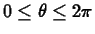and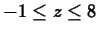. Use cylindrical coordinates.
2.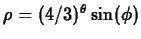forand. Use spherical coordinates.

3. Consider the functionforand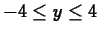, which looks like a deep valley with a mountain opposite it. Is it possible to find a path on the surface from the point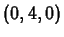to the point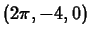such that the value ofis always between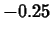and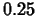? You do not have to find a formula for your path, but you must present convincing evidence that it exists. For example, you might sketch your path in by hand on an appropriate contour plot.

4. Plot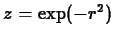forfirst using the implicitplot3d command and then using a parametric plot and the plot3d command.

5. Explain why a torus can't be represented in Cartesian coordinates as, in cylindrical coordinates as, or in spherical coordinates as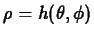.Next: About this document ... Up: lab_template Previous: lab_template
William W. Farr
2000-11-22